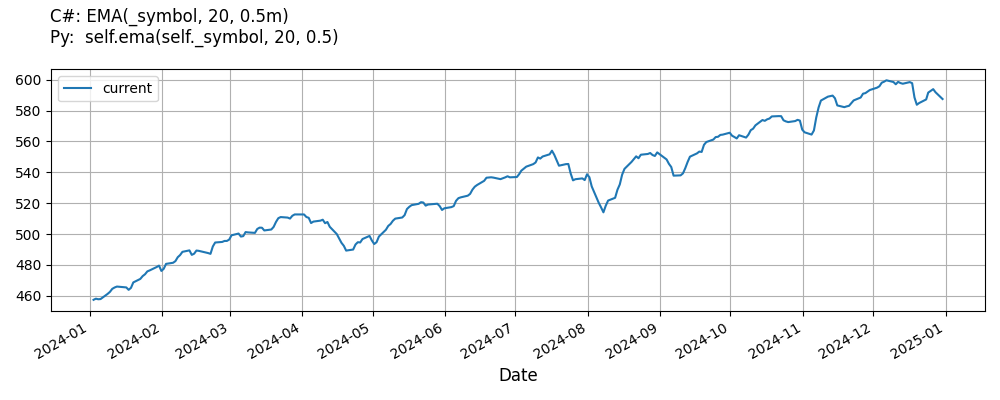# Supported Indicators

## Exponential Moving Average

### Introduction

This indicator represents the traditional exponential moving average indicator (EMA). When the indicator is ready, the first value of the EMA is equivalent to the simple moving average. After the first EMA value, the EMA value is a function of the previous EMA value. Therefore, depending on the number of samples you feed into the indicator, it can provide different EMA values for a single security and lookback period. To make the indicator values consistent across time, warm up the indicator with all the trailing security price history.

To view the implementation of this indicator, see the LEAN GitHub repository.

### Using EMA Indicator

To create an automatic indicators for ExponentialMovingAverage, call the EMA helper method from the QCAlgorithm class. The EMA method creates a ExponentialMovingAverage object, hooks it up for automatic updates, and returns it so you can used it in your algorithm. In most cases, you should call the helper method in the Initialize method.

public class ExponentialMovingAverageAlgorithm : QCAlgorithm
{
private Symbol _symbol;
private ExponentialMovingAverage _ema;

public override void Initialize()
{
_ema = EMA(_symbol, 20, 0.5);
}

public override void OnData(Slice data)
{
{
// The current value of _ema is represented by itself (_ema)
// or _ema.Current.Value
Plot("ExponentialMovingAverage", "ema", _ema);

}
}
}
class ExponentialMovingAverageAlgorithm(QCAlgorithm):
def Initialize(self) -> None:
self.ema = self.EMA(self.symbol, 20, 0.5)

def OnData(self, slice: Slice) -> None:
# The current value of self.ema is represented by self.ema.Current.Value
self.Plot("ExponentialMovingAverage", "ema", self.ema.Current.Value)



The following reference table describes the EMA method:

### EMA()1/2

            ExponentialMovingAverage QuantConnect.Algorithm.QCAlgorithm.EMA (
Symbol                           symbol,
Int32                            period,
*Nullable<Resolution>      resolution,
*Func<IBaseData, Decimal>  selector
)


Creates an ExponentialMovingAverage indicator for the symbol. The indicator will be automatically updated on the given resolution.

### EMA()2/2

            ExponentialMovingAverage QuantConnect.Algorithm.QCAlgorithm.EMA (
Symbol                           symbol,
Int32                            period,
Decimal                          smoothingFactor,
*Nullable<Resolution>      resolution,
*Func<IBaseData, Decimal>  selector
)


Creates an ExponentialMovingAverage indicator for the symbol. The indicator will be automatically updated on the given resolution.

If you don't provide a resolution, it defaults to the security resolution. If you provide a resolution, it must be greater than or equal to the resolution of the security. For instance, if you subscribe to hourly data for a security, you should update its indicator with data that spans 1 hour or longer.

You can manually create a ExponentialMovingAverage indicator, so it doesn't automatically update. Manual indicators let you update their values with any data you choose.

Updating your indicator manually enables you to control when the indicator is updated and what data you use to update it. To manually update the indicator, call the Update method with time/number pair, or an IndicatorDataPoint. The indicator will only be ready after you prime it with enough data.

public class ExponentialMovingAverageAlgorithm : QCAlgorithm
{
private Symbol _symbol;
private ExponentialMovingAverage _ema;

public override void Initialize()
{
_ema = new ExponentialMovingAverage(20, 0.5);
}

public override void OnData(Slice data)
{
if (data.Bars.TryGeValue(_symbol, out var bar))
{
_ema.Update(bar.EndTime, bar.Close);
}

{
// The current value of _ema is represented by itself (_ema)
// or _ema.Current.Value
Plot("ExponentialMovingAverage", "ema", _ema);

}
}
}
class ExponentialMovingAverageAlgorithm(QCAlgorithm):
def Initialize(self) -> None:
self.ema = ExponentialMovingAverage(20, 0.5)

def OnData(self, slice: Slice) -> None:
bar = slice.Bars.get(self.symbol)
if bar:
self.ema.Update(bar.EndTime, bar.Close)

# The current value of self.ema is represented by self.ema.Current.Value
self.Plot("ExponentialMovingAverage", "ema", self.ema.Current.Value)



To register a manual indicator for automatic updates with the security data, call the RegisterIndicator method.

public class ExponentialMovingAverageAlgorithm : QCAlgorithm
{
private Symbol _symbol;
private ExponentialMovingAverage _ema;

public override void Initialize()
{
_ema = new ExponentialMovingAverage(20, 0.5);
RegisterIndicator(_symbol, _ema, Resolution.Daily);
}

public override void OnData(Slice data)
{
{
// The current value of _ema is represented by itself (_ema)
// or _ema.Current.Value
Plot("ExponentialMovingAverage", "ema", _ema);

}
}
}
class ExponentialMovingAverageAlgorithm(QCAlgorithm):
def Initialize(self) -> None:
self.ema = ExponentialMovingAverage(20, 0.5)
self.RegisterIndicator(self.symbol, self.ema, Resolution.Daily)

def OnData(self, slice: Slice) -> None:
# The current value of self.ema is represented by self.ema.Current.Value
self.Plot("ExponentialMovingAverage", "ema", self.ema.Current.Value)



The following reference table describes the ExponentialMovingAverage constructor:

### ExponentialMovingAverage()1/4

            ExponentialMovingAverage QuantConnect.Indicators.ExponentialMovingAverage (
string  name,
int     period
)


Initializes a new instance of the ExponentialMovingAverage class with the specified name and period.

### ExponentialMovingAverage()2/4

            ExponentialMovingAverage QuantConnect.Indicators.ExponentialMovingAverage (
string   name,
int      period,
decimal  smoothingFactor
)


Initializes a new instance of the ExponentialMovingAverage class with the specified name and period.

### ExponentialMovingAverage()3/4

            ExponentialMovingAverage QuantConnect.Indicators.ExponentialMovingAverage (
int  period
)


Initializes a new instance of the ExponentialMovingAverage class with the default name and period.

### ExponentialMovingAverage()4/4

            ExponentialMovingAverage QuantConnect.Indicators.ExponentialMovingAverage (
int      period,
decimal  smoothingFactor
)


Initializes a new instance of the ExponentialMovingAverage class with the default name and period.

### Visualization

The following image shows plot values of selected properties of ExponentialMovingAverage using the plotly library.You can also see our Videos. You can also get in touch with us via Discord.